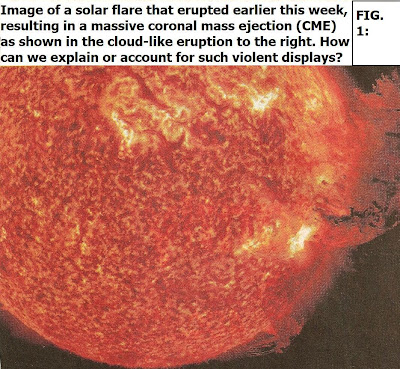## Thursday, June 9, 2011

### Accounting for Eruptive Solar Flares (1)Earlier this week the Sun erupted with unusual ferocity, with magnetic instability triggering a moderate solar flare that also incepted one of the largest coronal mass ejections on record. (See Fig. 1). How can such violent events be triggered, and say tied to specific unstable regions on the Sun? This has been an ongoing topic of my own research and I'd like to further explore it here.

To set the stage: I adopt a particularly simple model of a two-loop system, or two magnetic loops that interact over some time, call it dT. This model will always start with some initialized time, t0 and then incrementally increase, e.g. t1, t2, etc. to connform to discrete angular changes of one loop's orientation to the other's. The trick is to quantify how these angular changes occur in terms of the significant solar quantities.

One way (developed by DeMoulin, Pariat,. and Berger: 2006, Solar Phys., 233, 3) is to assign multiple valued angle functions, Θ_m. Since a lot will be based on these it helps to go through some background for those who may not be familiar with the math (though in many previous blogs we did do a lot with complex numbers, functions, and analyses!)

Each loop features opposite magnetic polarities (+, -) at its ends and we designate these in terms of B(+) and C(-) (for loop 1) and A(+) and A(-) for loop 2. Note that the position of the base footpoints – either B(+) or C(-) with respect to (A+,-) - can be described by x = rA-A, B-C(cos Θ, sin Θ). Following the lead of DeMoulin et al, 2006 (ibid.) in terms of method, one therefore needs a continuous function – without any branch cuts- denoted as a multiple valued function, Following the lead of DeMoulin et al, 2006 (ibid.) in terms of method, one therefore needs a continuous complex function – without any branch cuts- denoted as a multiple valued function, Θ_m .

The integration of the mutual helicity (£ BC, A) –magnetic free energy buildup in time then yields (see past blogs on magnetic helicity covered last year):

£ BC, A = { Θ_m(A+C)- - Θ_m(A+B+) + Θ_m (A-B+) - Θ_m (A-C-)}

In terms of interior angles (cf. DeMoulin et al, 2006) we may write:

£ARCH BC, A = 1/ 2π (a1 + a2)

where:

a1 = aA+ = [Θ_m (A+B+) - Θ_m (A+C-)]

a2 = aA- = [Θ_m (A-C-) - Θ_m (A-B+)]

where each a is actually 'alpha' the Greek letter, and is used to simplify notation.

I reiterate here that a1, 2 defines the angular extension of the loop segments as seen from the emerging flux points +,-A.

Using the principles of complex numbers, for which (by way of example):

Θ_m (A+C-) = Im log^m [(C -) - (A+)] and d log^m ( z)/ dz = 1/z

then we can also express the result for the mutual helicity of the system as:

£ BC, A = 1/ 2π Im log^m [(A-) - (B+)] [(C-)- (A+)] /[(B+) - (A+)] [(C-)- (A-)]

where the bracket makes use of the cross-ratio such that:

K(A,B,C,D) = (A – C) (B – D)/ (A – D) (B – C)

We now apply all this to the specific model depicted in Fig. 2 for two loops undergoing relative motions with respect to each other.

We take as starting values, estimated observed initial angular increments:

Θ_m (A+B+) = π/3

Θ_m(A+C-) = π/6

Θ_m(A-C-) = π/2

Θ_ m(A-B+) = π/4

This yields:

a1 = aA+ = [π/3 - π/6] = π/6

a2 = aA- = [π/2 - π/4] = π/4

and:

£ARCH BC, AA’ = 1/ 2π (a1 + a2) = (π/6 + π/4)/ 2π

and: £ARCH BC, AA’ = 5/ 24

Again, this refers to the relative helicity at the stages identified for the angular increments. We now inquire as to the possibility that a high temperature turbulent current sheet (“HTTCS”) could form and incept eruptive flares, say with the potential to produce eruptive prominences or CMEs. Research by Somov et al (1998) shows this is feasible in a quadripolar-type system such as illustrated (Fig. 2) They note that if reconnecting field lines (f1, f2 etc) become shorter, accelerated electron beams can arrive at the chromosphere faster. Field line length changes in the system used will disclose definite effects on the parameters, angle functions etc.

For example, with reference to Fig. 2, let field line f1 connect loop polarities (-A) and (+A) while field line f2 connects polarities (+B) and (-C). For our purposes we can assume these foot point “poles” bear a homologous relation to the “magnetic charges” used by Somov et al(see their Fig. 2), as follows:

(+B) <-> e(N)

(-C) <-> e(S)

+A <-> e(n)

-A <-> e(s)

Now, we know:

aA+ = [Θ_m (A+B+) - Θ_m (A+C-)]

and also:

aB+ = [Θ_m (B+A-) - Θ_m (C-A-)]

To fix ideas, we invoke a hypothetical scenario for which f2 changes relative to f1 such that the angular evolution occurs as depicted in the accompanying table (see graphic), where the t_j are spaced at 1000s intervals.

The initial (t0) angle displacements are:

aA+ = [1.6π/2 - π/3] = 1.4π/3

aB+ = [π/2 - π/3] = π/6

And by time (t2):

aA+ = [1.2π/2 - π/3] = 0.8π/3

aB+ = [1.4π/2 - π/3] = 1.1π/3

which change clearly shows foot points B+, A’ decreasing.

A key barometer is the angle (aB+) which increases in the model from π/6 (30 deg) to 0.8π/3 (48 deg) between t0 and t2. This increase in the angle aB+ translates into a decrease in the footpoint distance (B+- A’) and seems to conform to the more impulsive case reviewed by Somov et al (1998).

If we can verify other key quantitative factors, i.e. that the mutual helicity significantly increases, then it may be possible to show the energy is present for a highly eruptive flare event.

(To be continued)